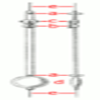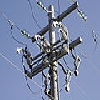#### You may also like### Differential Equation Matcher

Match the descriptions of physical processes to these differential equations.### The Not-so-simple Pendulum 2

Things are roughened up and friction is now added to the approximate simple pendulum### Differential Electricity

As a capacitor discharges, its charge changes continuously. Find the differential equation governing this variation.

# The Real Hydrogen Atom

### Why do this problem?

This problem provides a glimpse into the way differential equations develop at university both in terms of mathematical structure and also in their applications. It is ideal for high achieving maths students coming to the end of their school careers who wish to draw their learning together or to prepare themselves for the university challenges ahead.

### Possible approach

This problem is most suitable for the highest attaining mathematics students or those with a very strong interest in pursuing mathematical physics.

One thing might strike students: the problem involves a lot of reading and several parts to consider and solve. Encourage those working on it to read each part carefully and consider the mathematical ideas raised: the thinking about the issues can be of as much importance as doing the problem; reflecting on the solution obtained rather than simply getting the algebra correct is crucial! Furthermore, students who have become accustomed to completely solving problems might need to be reminded that partial solutions are acceptable and that at higher educational levels complete solutions and not the norm.

It is often a good idea to encourage students to talk about their mathematical experiences. Perhaps this problem could be given to students who consider the problem with plenty of thinking space and then give a short presentation on the problem to the rest of the group at a later date. Alternatively, small groups of interested students could consider the problem collectively and try to make sense of the ideas, being proactive in helping themselves out of difficulty.

### Key questions

The problem is well structured into different parts, so could be attempted by students independently. Some good questions to help students out of difficulty are:

Are you clear as to what is constant and what is variable in each case?

Have you understood the meaning of the coordinate system?

Have you understood the meaning of the variables?

Do you have a plan: do you know what you are trying to solve? What sort of maths might help you to solve it?

### Possible extension

There is plenty of extension built into this problem. Interested students might also try more of the problems from the Advanced Scientific Mathematics section of stemNRICH.

### Possible support

There are many more straightforward differential equation problems on the Advanced Scientific Mathematics section of stemNRICH.